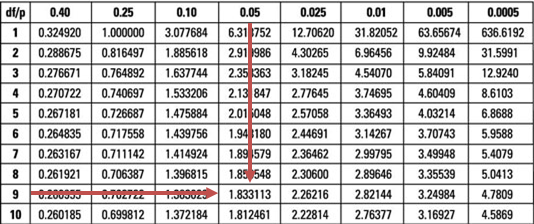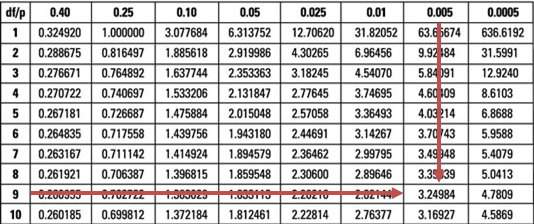Limited Time Offer: Save 10% on all 2021 and 2022 Premium Study Packages with promo code: BLOG10    Select your Premium Package »Uses of the t-test and the z-test

The z-test

The z-test is the ideal hypothesis test to conduct in a normal distribution of a random variable. In addition, the variance of the population must be known. The z-statistic refers to the test statistic computed for hypothesis testing.

Testing H0: μ = μ0 using the z-test

Given a random sample of size n from a normally distributed population with mean μ and variance σ2, and a sample mean X̄, we can compute the z-statistic as:

$$\text z-\text{statistic} =\cfrac {(\bar{X} – \mu_0)}{\left(\frac {\sigma}{\sqrt n} \right)}$$

Where:

$$\bar{X}$$ is the sample mean.

$$μ_0$$ is the hypothesized mean of the population.

$$σ$$ is the standard deviation of the population.

$$n$$ is the sample size.

Once computed, the z-statistic is compared to the critical value that corresponds to the level of significance of the test. For example, if the significance level is 5%, the z-statistic is screened against the upper or lower 95% point of the normal distribution (±1.96). The decision rule is to reject the H0 if the z-statistic falls within the critical or rejection region.

Example: z-test

Academics carried out a study on 50 former United States presidents and found an average IQ of 135. You are required to carry out a 5% statistical test to determine whether the average IQ of presidents is greater than 130. (IQs are distributed normally, and previous studies indicate that σ = 25.)

Solution

First, you have to state the hypothesis:

H0: μ = 130

H1: μ > 130

Assuming the H0 is true, $$\frac {(\bar{X} – 130)}{\left(\frac {\sigma}{\sqrt n} \right)} \sim N(0,1)$$.

The z-statistic is $$\cfrac {(135 – 130)}{\left(\frac {25}{\sqrt {50}} \right)} = 1.414$$.

This is a right-tailed test. Therefore, we compare our test statistic to the upper 95% point of the standard normal distribution (1.6449). Since 1.414 is less than 1.6449, we do not have sufficient evidence to reject the H0. As such, it would be reasonable to conclude that the average IQ of U.S. presidents is not more than 130.

The t-test

The t-test is based on the t-distribution. The test is appropriate for testing the value of a population mean when:

• σ is unknown; and
• The sample size is large (n ≥ 30), and if n < 30, the distribution must either be normal or approximately normal.

Testing H0: μ = μ0 Using the t-test

We compute a t-statistic with n – 1 degrees of freedom as:

$$t_{n-1} = \cfrac {(\bar{X} – \mu_0)}{\left(\frac {s}{\sqrt n} \right)}$$

Where:

$$\bar{X}$$ is the sample mean.

$$μ_0$$ is the hypothesized mean of the population.

$$s$$ is the standard deviation of the sample.

$$n$$ is the sample size.

Example: t-test

The annual rate of rainfall (cm) in a certain equatorial country over the last 10 years is given below:

{ 25   26   25    27   28   29   28   27   26   25 }

Financial analysts in the country wish to determine whether the average rate of rainfall has increased from its former value of 23. Carry out a statistical test at the 5% level.

Solution

As always, you should begin by stating the hypothesis:

H0: μ ≤ 23

H1: μ > 23

If we assume that the annual rainfall quantities are distributed normally and recorded independently, then:

$$\cfrac {(\bar{X} – 23)}{\left(\frac {S}{\sqrt n} \right)} \sim t_{n- 1}$$

Please, confirm that X̄ = 26.6 and S = 1.43.

Therefore, our t-statistic = $$\cfrac {(26.6 – 23)}{\left(\frac {1.43}{\sqrt {10}}\right)} = 7.96$$.

Our test statistic (7.96) is greater than the upper 95% point of the t0.05,9 distribution (1.833).Therefore, we have sufficient evidence to reject the H0. As such, it is reasonable to conclude that the average annual rainfall has increased from its former long-term average of 23.

Question

What is the value of t in the example above if the level of significance is reduced from 5% to 0.5%, and does this change the decision rule?

1. 2.02; it does not change the decision rule.
2. 3.25; it does not change the decision rule.
3. 3.25; it does change the decision rule.

Solution

A quick glance at the t0.005,9 distribution when α = 0.5% gives a value of 3.25.However, the evidence against the H0 is too strong since our test statistic (7.96) is still greater than 3.25. As such, the conclusion would remain unchanged.

(Note to candidates: You might also work out the solutions to the above examples and questions using p-values instead of critical values. The decision rules would remain unchanged provided you work out the p-values correctly.)

Featured Study with UsCFA® Exam and FRM® Exam Prep Platform offered by AnalystPrep

Study Platform

Learn with Us

Subscribe to our newsletter and keep up with the latest and greatest tips for success
Online TutoringOur videos feature professional educators presenting in-depth explanations of all topics introduced in the curriculum.

Video LessonsSergio Torrico
2021-07-23
Excelente para el FRM 2 Escribo esta revisión en español para los hispanohablantes, soy de Bolivia, y utilicé AnalystPrep para dudas y consultas sobre mi preparación para el FRM nivel 2 (lo tomé una sola vez y aprobé muy bien), siempre tuve un soporte claro, directo y rápido, el material sale rápido cuando hay cambios en el temario de GARP, y los ejercicios y exámenes son muy útiles para practicar.diana
2021-07-17
So helpful. I have been using the videos to prepare for the CFA Level II exam. The videos signpost the reading contents, explain the concepts and provide additional context for specific concepts. The fun light-hearted analogies are also a welcome break to some very dry content. I usually watch the videos before going into more in-depth reading and they are a good way to avoid being overwhelmed by the sheer volume of content when you look at the readings.Kriti Dhawan
2021-07-16
A great curriculum provider. James sir explains the concept so well that rather than memorising it, you tend to intuitively understand and absorb them. Thank you ! Grateful I saw this at the right time for my CFA prep.nikhil kumar
2021-06-28
Very well explained and gives a great insight about topics in a very short time. Glad to have found Professor Forjan's lectures.Marwan
2021-06-22
Great support throughout the course by the team, did not feel neglectedBenjamin anonymous
2021-05-10
I loved using AnalystPrep for FRM. QBank is huge, videos are great. Would recommend to a friendDaniel Glyn
2021-03-24
I have finished my FRM1 thanks to AnalystPrep. And now using AnalystPrep for my FRM2 preparation. Professor Forjan is brilliant. He gives such good explanations and analogies. And more than anything makes learning fun. A big thank you to Analystprep and Professor Forjan. 5 stars all the way!michael walshe
2021-03-18
Professor James' videos are excellent for understanding the underlying theories behind financial engineering / financial analysis. The AnalystPrep videos were better than any of the others that I searched through on YouTube for providing a clear explanation of some concepts, such as Portfolio theory, CAPM, and Arbitrage Pricing theory. Watching these cleared up many of the unclarities I had in my head. Highly recommended.